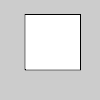# Py5Shape.vertex()#

Add a new vertex to a `Py5Shape` object.

## Examples#```def setup():
s = py5.create_shape()
s.begin_shape()
s.vertex(20, 80)
s.vertex(80, 80)
s.vertex(50, 20)
s.end_shape(py5.CLOSE)

py5.shape(s)
``````def setup():
py5.size(100, 100, py5.P3D)
# drawing vertices in 3D requires P3D
# as a parameter to size()
s = py5.create_shape()
s.begin_shape()
s.vertex(30, 20, 10)
s.vertex(85, 20, 10)
s.vertex(85, 75, 10)
s.vertex(30, 75, 10)
s.end_shape(py5.CLOSE)
py5.shape(s)
``````def setup():
py5.size(100, 100, py5.P2D)
# call py5.texture_mode() here to inherit mode setting
# py5.texture_mode(py5.NORMAL)
s = py5.create_shape()
s.begin_shape()
s.texture(img)
s.texture_mode(py5.NORMAL)
s.vertex(20, 20, 0, 0)
s.vertex(20, 80, 0, 1)
s.vertex(80, 80, 1, 1)
s.vertex(80, 20, 1, 0)
s.end_shape(py5.CLOSE)

py5.shape(s)
```

## Description#

Add a new vertex to a `Py5Shape` object. All shapes are constructed by connecting a series of vertices. The `vertex()` method is used to specify the vertex coordinates for points, lines, triangles, quads, and polygons. It is used exclusively within the Py5Shape.begin_shape() and Py5Shape.end_shape() methods.

Drawing a vertex in 3D using the `z` parameter requires the `P3D` renderer, as shown in the second example.

This method is also used to map a texture onto geometry. The Py5Shape.texture() function declares the texture to apply to the geometry and the `u` and `v` coordinates define the mapping of this texture to the form. By default, the coordinates used for `u` and `v` are specified in relation to the image’s size in pixels, but this relation can be changed with the `Py5Shape` object’s Py5Shape.texture_mode() method or by calling the Sketch’s texture_mode() method before the shape is created.

Underlying Processing method: PShape.vertex

## Signatures#

```vertex(
x: float,  # x-coordinate of the vertex
y: float,  # y-coordinate of the vertex
/,
) -> None

vertex(
x: float,  # x-coordinate of the vertex
y: float,  # y-coordinate of the vertex
u: float,  # horizontal coordinate for the texture mapping
v: float,  # vertical coordinate for the texture mapping
/,
) -> None

vertex(
x: float,  # x-coordinate of the vertex
y: float,  # y-coordinate of the vertex
z: float,  # z-coordinate of the vertex
/,
) -> None

vertex(
x: float,  # x-coordinate of the vertex
y: float,  # y-coordinate of the vertex
z: float,  # z-coordinate of the vertex
u: float,  # horizontal coordinate for the texture mapping
v: float,  # vertical coordinate for the texture mapping
/,
) -> None
```

Updated on September 01, 2022 16:36:02pm UTC